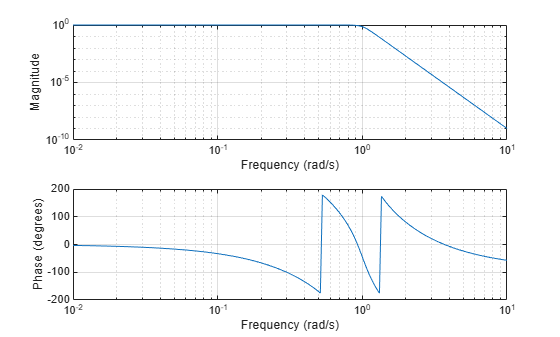buttap

Butterworth filter prototype

Description

example

[z,p,k] = buttap(n) returns the poles and gain of an order n Butterworth analog lowpass filter prototype.

Examples

collapse all

Design a 9th-order Butterworth analog lowpass filter. Display its magnitude and phase responses.

[z,p,k] = buttap(9);          % Butterworth filter prototype
[num,den] = zp2tf(z,p,k);     % Convert to transfer function form
freqs(num,den)                % Frequency response of analog filterInput Arguments

collapse all

Order of Butterworth filter, specified as a positive integer scalar.

Output Arguments

collapse all

Zeros of the system, returned as a matrix. z contains the numerator zeros in its columns. z is an empty matrix because there are no zeros.

Poles of the system, returned as a column vector. p contains the pole locations of the denominator coefficients of the transfer function.

Gains of the system, returned as a scalar. k contains the gains for each numerator transfer function.

Algorithms

The function buttap returns the poles in the length n column vector p and the gain in scalar k. z is an empty matrix because there are no zeros. The transfer function is

$H\left(s\right)=\frac{z\left(s\right)}{p\left(s\right)}=\frac{k}{\left(s-p\left(1\right)\right)\left(s-p\left(2\right)\right)\cdots \left(s-p\left(n\right)\right)}$

z = [];
p = exp(sqrt(-1)*(pi*(1:2:2*n-1)/(2*n)+pi/2)).';
k = real(prod(-p));

Note

The function buttap returns zeros, poles, and gain (z, p, and k) in MATLAB®. However, the generated C/C++ code for buttap returns only poles p and gain k since zeros z is always an empty matrix.

Butterworth filters are characterized by a magnitude response that is maximally flat in the passband and monotonic overall. In the lowpass case, the first 2n-1 derivatives of the squared magnitude response are zero at ω = 0. The squared magnitude response function is

${|H\left(\omega \right)|}^{2}=\frac{1}{1+{\left(\omega /{\omega }_{0}\right)}^{2n}}$

corresponding to a transfer function with poles equally spaced around a circle in the left half plane. The magnitude response at the cutoff angular frequency ω0 is always $1/\sqrt{2}$ regardless of the filter order. buttap sets ω0 to 1 for a normalized result.

 Parks, T. W., and C. S. Burrus. Digital Filter Design. New York: John Wiley & Sons, 1987.